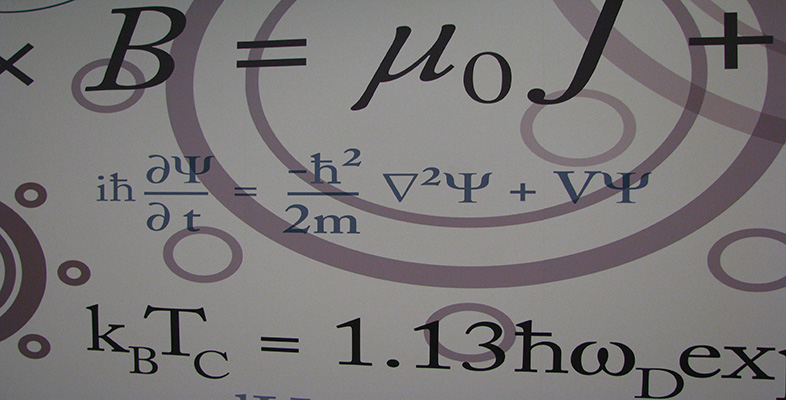Number systems

This free course is available to start right now. Review the full course description and key learning outcomes and create an account and enrol if you want a free statement of participation.

Free course

Examples

• 1.   The condition ‘is equal to’ is a relation on any set of real numbers because, for any x, y in the set, the statement ‘x is equal to y’ is either definitely true or definitely false. This relation is usually denoted by the symbol =. For this relation, each real number in the set is related only to itself!

• 2.   The condition ‘is less than’ is a relation on any set of real numbers, and we usually denote it by the symbol <. For example, −2 < 1, but 1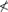−2 and 33.

• 3.   The condition ‘is the derivative of’ is a relation on any set of functions. We can define

•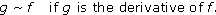• For example, let f(x) = x3, g(x) = 3x2 and h(x) = 2ex. Then gf because g is the derivative of f, and hh because h is the derivative of h, but f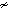g because f is not the derivative of g.

• 4.   On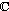, we can define a relation

•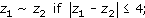• that is, z1 is related to z2 if the distance between z1 and z2 in the complex plane is less than or equal to 4. For example, 1 + i2 − i because

•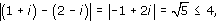• but 1 + i3+ 5i because

•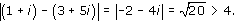M208_6